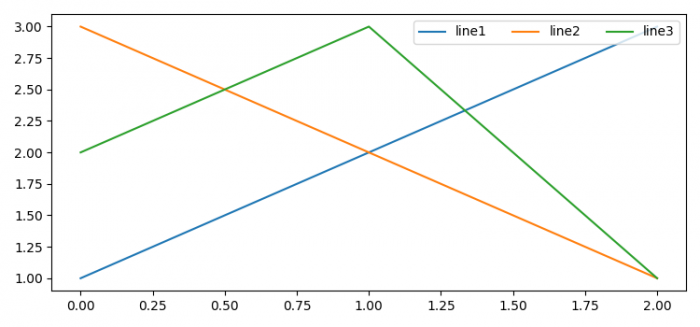# How to show legend elements horizontally in Matplotlib?

To show legend elements horizontally, we can take the following steps

• Set the figure size and adjust the padding between and around the subplots.
• Using plot() method, plot lines with the labels line1, line2 and line3.
• Place a legend on the figure using legend() method, with number of labels for ncol value in the argument.
• To display the figure, use show() method.

## Example

from matplotlib import pyplot as plt

plt.rcParams["figure.figsize"] = [7.50, 3.50]
plt.rcParams["figure.autolayout"] = True

line1, = plt.plot([1, 2, 3], label="line1")
line2, = plt.plot([3, 2, 1], label="line2")
line3, = plt.plot([2, 3, 1], label="line3")
plt.legend(ncol=3, loc="upper right")
plt.show()

## Output